Ex 13.2

Chapter 13 Class 11 Limits and Derivatives
Serial order wise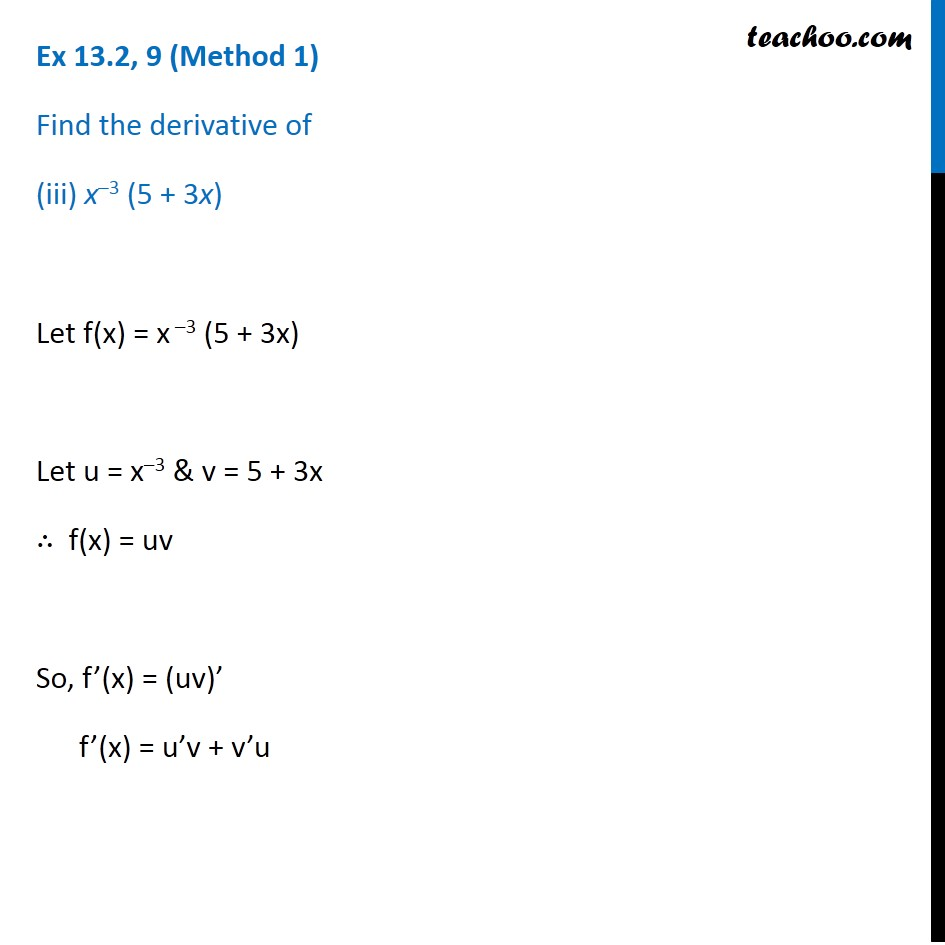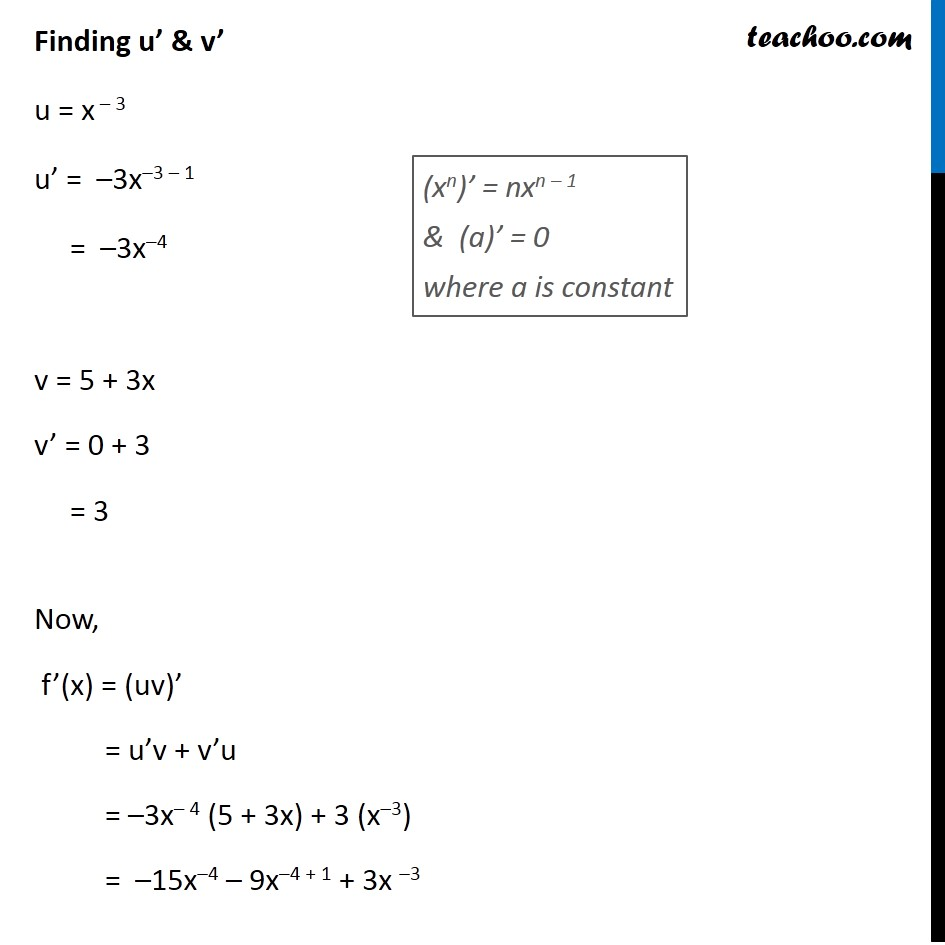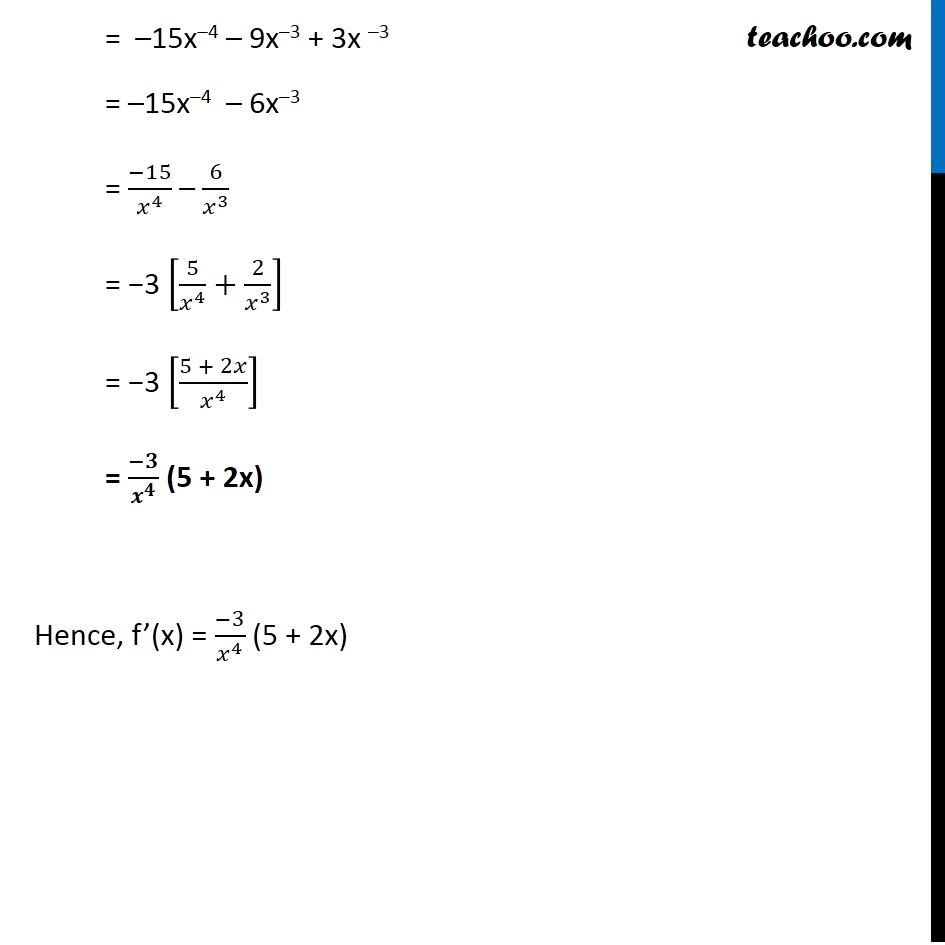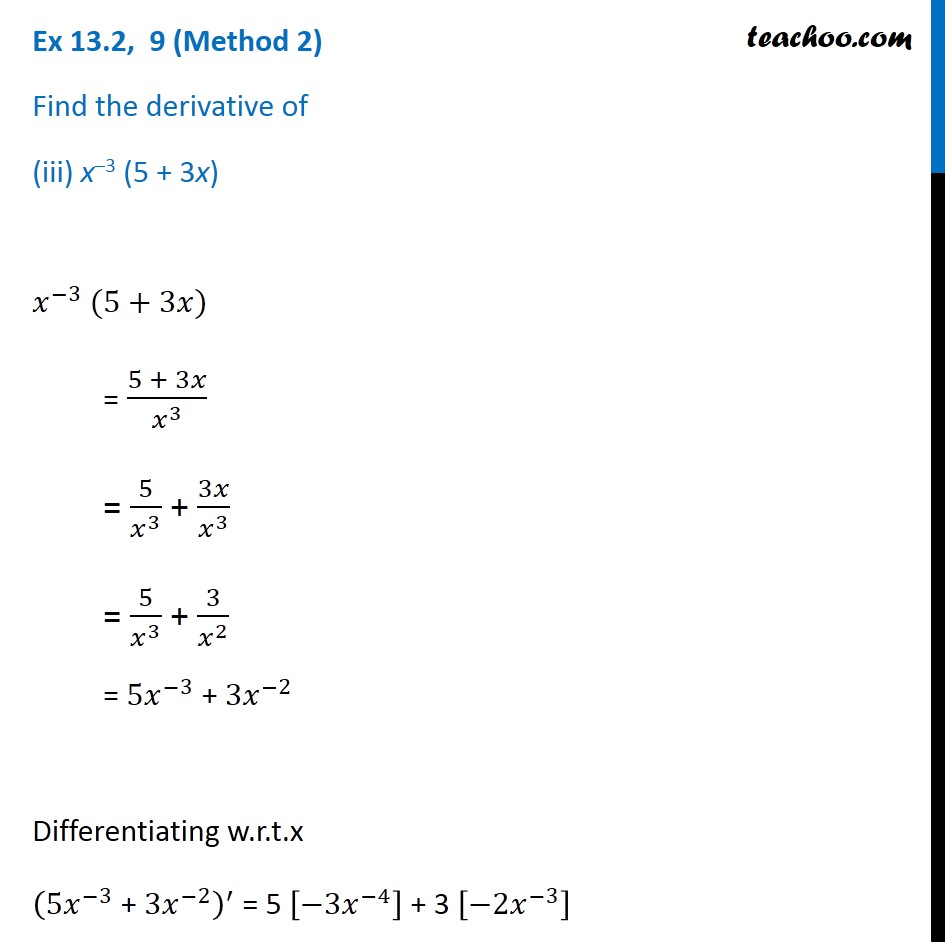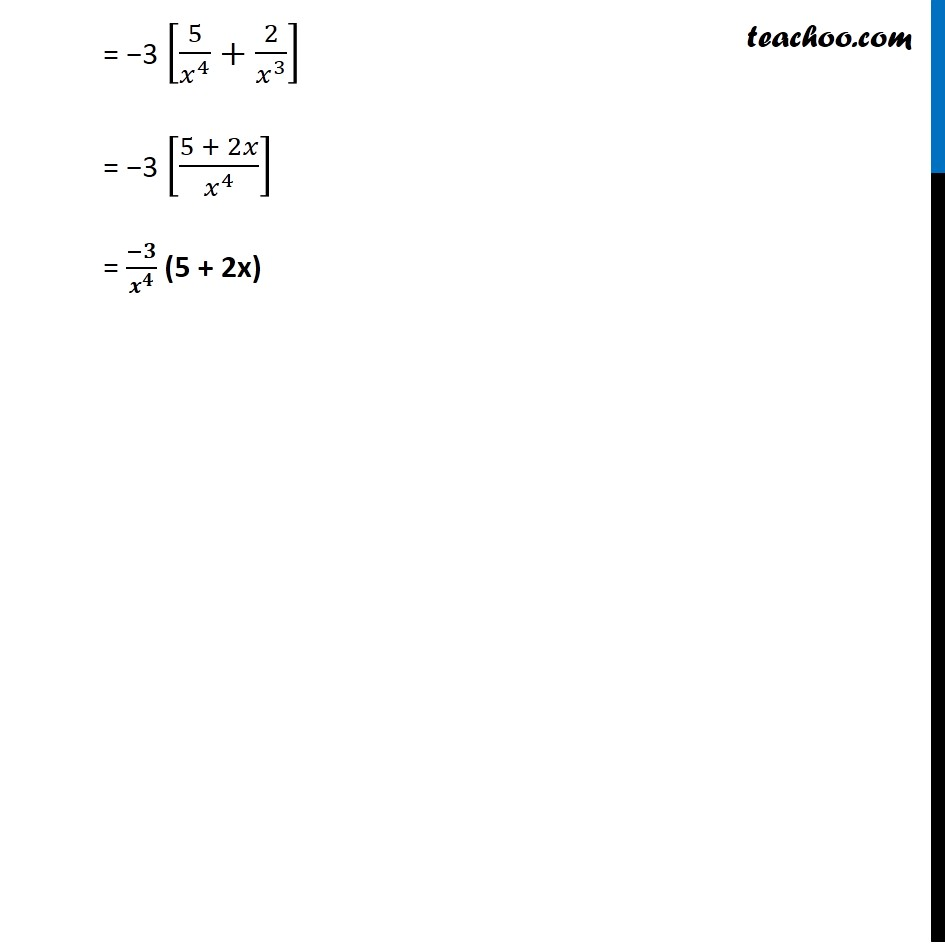Maths Crash Course - Live lectures + all videos + Real time Doubt solving!

### Transcript

Ex 13.2, 9 (Method 1) Find the derivative of (iii) x–3 (5 + 3x) Let f(x) = x –3 (5 + 3x) Let u = x–3 & v = 5 + 3x ∴ f(x) = uv So, f’(x) = (uv)’ f’(x) = u’v + v’u Finding u’ & v’ u = x – 3 u’ = –3x–3 – 1 = –3x–4 v = 5 + 3x v’ = 0 + 3 = 3 Now, f’(x) = (uv)’ = u’v + v’u = –3x– 4 (5 + 3x) + 3 (x–3) = –15x–4 – 9x–4 + 1 + 3x –3 (xn)’ = nxn – 1 & (a)’ = 0 where a is constant = –15x–4 – 9x–3 + 3x –3 = –15x–4 – 6x–3 = (−15)/𝑥^4 – 6/𝑥^3 = −3 [5/𝑥^4 +2/𝑥^3 ] = −3 [(5 + 2𝑥)/𝑥^4 ] = (−𝟑)/𝒙^𝟒 (5 + 2x) Hence, f’(x) = (−3)/𝑥^4 (5 + 2x) Ex 13.2, 9 (Method 2) Find the derivative of (iii) x–3 (5 + 3x) 𝑥^(−3) (5+3𝑥) = (5 + 3𝑥)/𝑥^3 = 5/𝑥^3 + 3𝑥/𝑥^3 = 5/𝑥^3 + 3/𝑥^2 = 〖5𝑥〗^(−3) + 〖3𝑥〗^(−2) Differentiating w.r.t.x (〖5𝑥〗^(−3) " + " 〖3𝑥〗^(−2) )^′ = 5 [−3𝑥^(−4) ] + 3 [−2𝑥^(−3) ] = −3 [5/𝑥^4 +2/𝑥^3 ] = −3 [(5 + 2𝑥)/𝑥^4 ] = (−𝟑)/𝒙^𝟒 (5 + 2x)